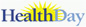# Men's Health# Determining Body Mass Index for Children and Teens

## What is body mass index?

Determining how much your child or teen should weigh isn't a simple matter of looking at an insurance height-weight chart. It includes considering the amount of bone, muscle, and fat in their body. The amount of fat is the critical measurement.

A good indicator of how much fat your child or teen carries is the body mass index (BMI). Although it's not a perfect measure, it gives an indirect assessment of how much of your teen's body is composed of fat. BMI is based on weight and height. As a result, it's only a gauge of body fatness. Two people may have the exact same BMI, yet have different amounts of actual body fat.

The formulas below apply to adults only. For children and teens ages 2 to 19 years, the BMI varies by age and sex. An additional step must be done after the BMI has been determined using one of the formulas below. The BMI-for-age percentile is determined by comparing your child or teen's weight to that of other children or teens of the same age and sex.

In other words, by plotting your child's BMI value into the  CDC's BMI-for-age growth chart , you can determine if your child is underweight (below the 5th percentile), within normal range (5th to 85th percentile), overweight (85th to 95th percentile), or obese (at or above the 95th percentile). Or you can easily calculate it from the CDC's online tool

## To calculate an adult's BMI using the English formula

A BMI between 25 to 29.9 is considered overweight. Anything at or above 30 is considered obese. Normal weight is a BMI is between 18.5 and 24.9. BMI cut-offs for people of South Asian descent are different.

BMI can be calculated using pounds and inches:

Multiply your weight in pounds by 703.

For example, a person who weighs 165 pounds and is 5 feet 4 inches (64 inches) tall has a BMI of 28. Multiply 165 by 703 for a total of 115,995. Divide that by 64 for a total of 1812. Divide that total by 64 for a BMI of 28..

## To calculate an adult's BMI using the metric formula

BMI can be calculated using kilograms and meters: